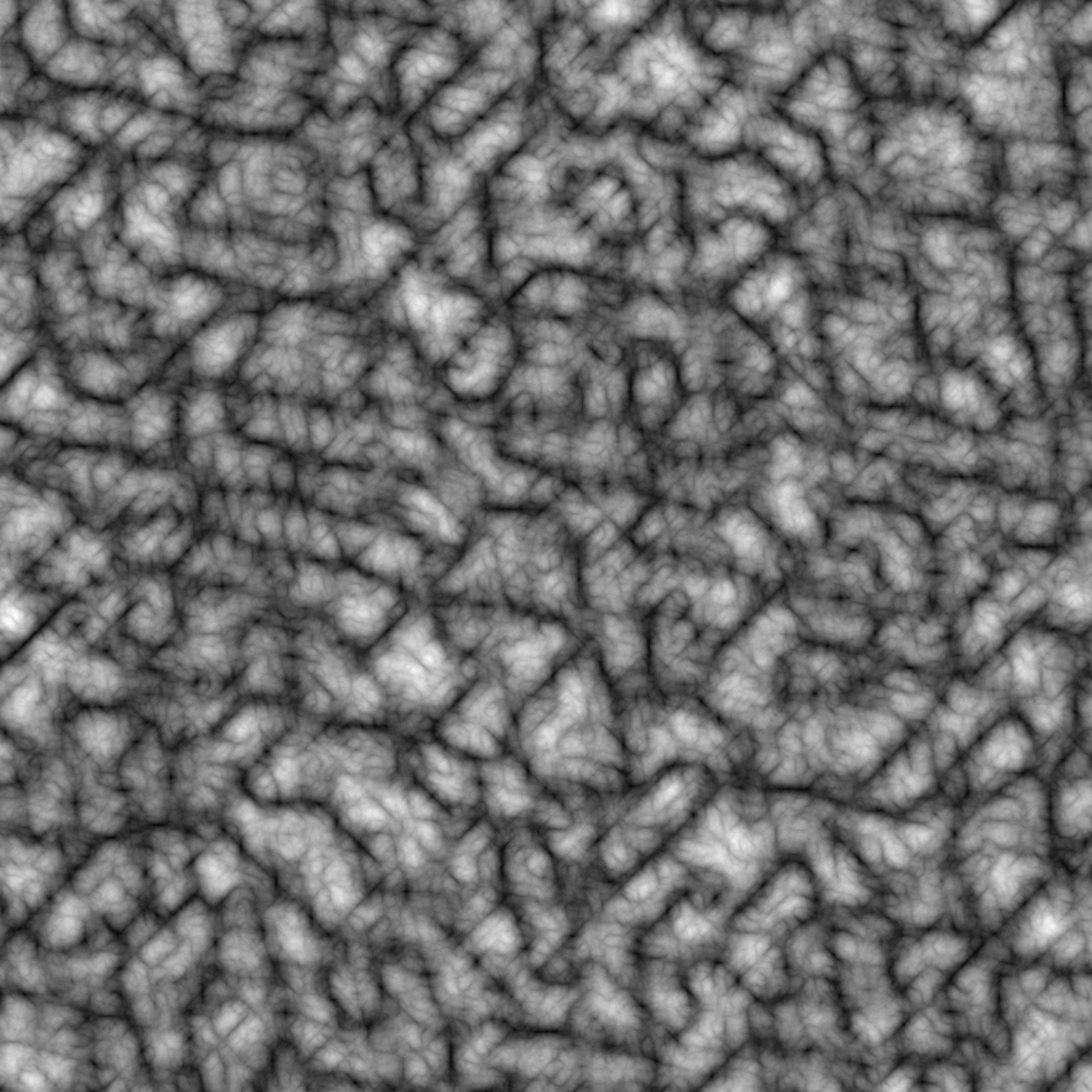The billow fractal is a slight modification of the fbm() fractal. Before adding the new layer onto the last, the new layer is modified by taking the absolute value, multiplying by 2, and subtracting one. The result is that the new value will not contain negative values and so will always add on top of the old values. This function is intended to be used in conjunction with fracture()

billow(base, new, strength, ...)

## Arguments

base The prior values to modify The new values to modify base with A value to modify new with before applying it to base ignored

Other Fractal functions: clamped(), fbm(), ridged()
grid <- long_grid(seq(1, 10, length.out = 1000), seq(1, 10, length.out = 1000))
grid$simplex <- fracture(gen_simplex, billow, octaves = 8, x = grid$x,